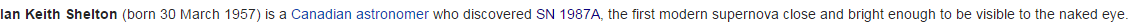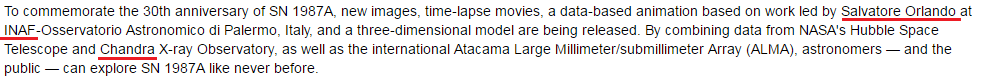CipherFeb 24, 2017

Supernova 1987A, Saturn/Pi coding of Supernova bullshit (do they really exist in reality?)

Here is the link to NASA's article that came out on 2/24/2017 - The Dawn of a New Era for Supernova 1987a
This story is a prime example of propaganda by the numbers, and these people actually call themselves scientistsFirst I should point out that they are bringing this up after 30th anniversary of discovery of this "Supernova" (in astrology Saturn's return takes 30 years (360 months) a lot like full circle (360°), it seems like these people are doing everything by the numbers of Saturn) - 30 years is 10,957 days (1331st prime number is 10,957 (11x11x11 = 1331) - 13 and 31 are also numbers associated with Saturn)
Three decades = 7+19+9+22+22 + 23+22+24+26+23+22+8 = 227 (Reverse Ordinal)
Note how their article starts with "Three decades" which has gematria connecting to circle (22/7 = 3.14), the coding of Pi and "inverse Pi" is very frequent with NASA and all other parts of mainstream
The supernova is called "1987a" which also connects to the circle which is the reason it's being reported by these numbers
Nineteen eighty seven A = 13+18+13+22+7+22+22+13 + 22+18+20+19+7+2 + 8+22+5+22+13 + 26 = 314 (Reverse Ordinal)
Twenty two divided by seven = 20+23+5+14+20+25 + 20+23+15 + 4+9+22+9+4+5+4 + 2+25 + 19+5+22+5+14 = 314 (Ordinal)This is a picture from the video in this article (Video link) , notice the 8 behind the circle of "Supernova". 8 is the symbol for infinity and also in astrology Saturn is the ruler of number 8 (note that 3.14 reduces to 8 as well)So this supernova was supposedly found on 2/23/1987 in the time of Pisces = 44 (S exception), Space = 44.... 2/23 stands for 223, a number coded over and over in mainstream media (Masonic = 223 Jewish, The Synagogue of Satan = 223, also 223 shows up in Revelation 13:18 where the number of man/beast (666) comes up)

Another reason for tor this Supernova to have Pi coding is the date numerology of it's "discovery"
2/23/1987 - 2+2+3 + 1+9+8+7 = 32Circle = 3+9+9+3+3+5 = 32 (Reduced)
2/23/1987 - (2) + (23) + 1+9+8+7 = 50Circle = 3+9+18+3+12+5 = 50 (Ordinal), Thirty two = 2+8+9+9+2+7 + 2+5+6 = 50 (Reduced)
2/23/1987 - (2) + (23) + (19) + (87) = 131 Supernova = 19+21+16+5+18+14+15+22+1 = 131 (Ordinal) - 32nd prime number is 131
2/23/87 - (2) + (23) + (87) = 112 - Supernova = 8+6+11+22+9+13+12+5+26 = 112 (Reverse Ordinal)
Circle = 24+18+9+24+15+22 = 112 (Reverse Ordinal)
Twenty third of February = 2+5+5+5+2+7 + 2+8+9+9+4 + 6+6 + 6+5+2+9+3+1+9+7 = 112 (Reduced)
Could there be a better date for the "discovery of Supernova"??
This coding is absolutely ridiculous... This is what science has become - pure propaganda by the numbers... Every part of society has been infiltrated by these spineless mongoloids and they make sure to put their fingerprint on everything you can find.I can't find anything for Oscar Duhalde
Shelton = 8+1+4+6+7+3+4 = 33 (Reverse Reduced)
Shelton = 19+8+5+12+20+15+14 = 93 (Ordinal)Notice that he's born on 3/30 and he discovered this "Supernova" 330 days after his birthdaySalvatore Orlando = 1+1+3+4+1+2+6+9+5 + 6+9+3+1+5+4+6 = 66 (Reduced)
INAF = 9+50+1+6 = 66 (English)
Thirty three = 2+8+9+9+2+7 + 2+8+9+5+5 = 66 (Reduced)

Chandra = 3+8+1+40+4+80+1 = 137 (Jewish) - 33rd prime number is 137
The significance of 137 probably comes from Golden Angle which can be seen in the way plants grow their leavesHere's a link that goes into all of that good stuff - Golden proportion in nature

There's some more stuff to this bullshit story but there's no reason to decode it any further since I already covered the key points.